Question

A construction zone on a highway has a posted speed limit of 40 miles per hour. The speeds of vehicles passing through this construction zone are normally distributed with a mean of 46 miles per hour and a standard deviation of 4 miles per hour.

A. The fastest 10% of the vehicles passing through this construction zone are going at what speed?

A) z score corresponding to top 10% area = 1.28

Hence,

Fastest 10% vehicle's speed = 46 + 1.28*4 = 51.12 miles per hour

#### Earn Coins

Coins can be redeemed for fabulous gifts.

Similar Homework Help Questions
• ### 6. A construction zone on a highway has a posted speed limit of 40 Kilometers per...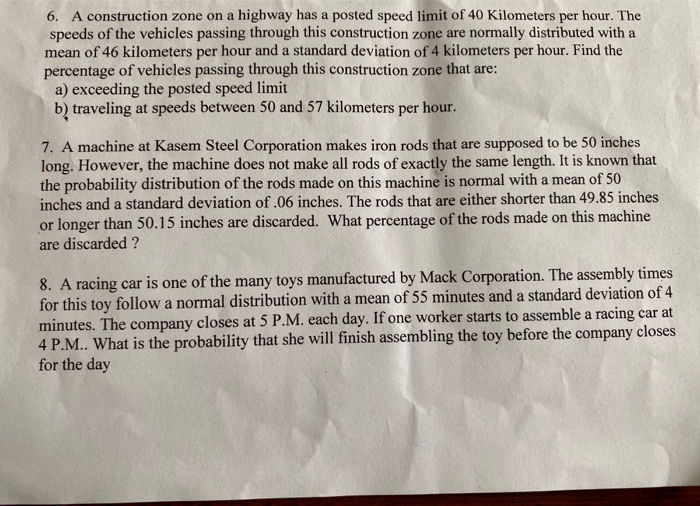6. A construction zone on a highway has a posted speed limit of 40 Kilometers per hour. The speeds of the vehicles passing through this construction zone are normally distributed with a mean of 46 kilometers per hour and a standard deviation of 4 kilometers per hour. Find the percentage of vehicles passing through this construction zone that are: a) exceeding the posted speed limit b) traveling at speeds between 50 and 57 kilometers per hour. 7. A machine at...

• ### Let x be a continuous random variable that is normally distributed with a mean of 25...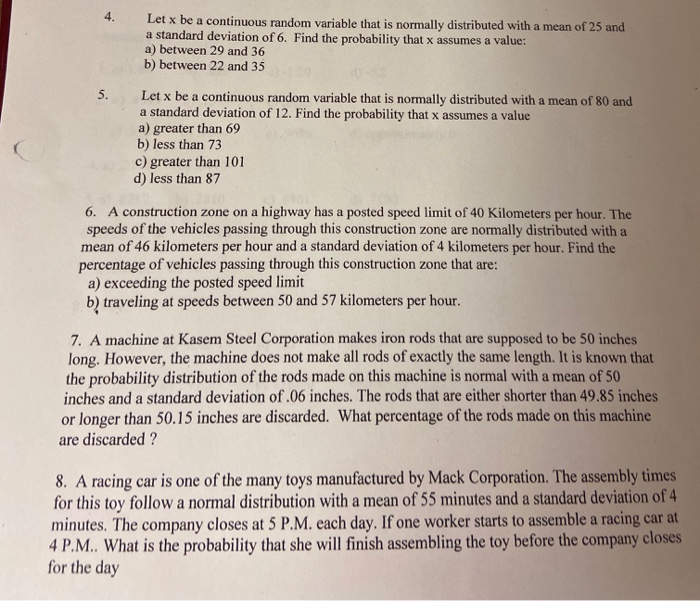Let x be a continuous random variable that is normally distributed with a mean of 25 and a standard deviation of 6. Find the probability that x assumes a value: a) between 29 and 36 b) between 22 and 35 Let x be a continuous random variable that is normally distributed with a mean of 80 and a standard deviation of 12. Find the probability that x assumes a value a) greater than 69 b) less than 73 c) greater...

• ### 4. (12 pts) The speed limit on a highway is 70 miles per hour. A sample...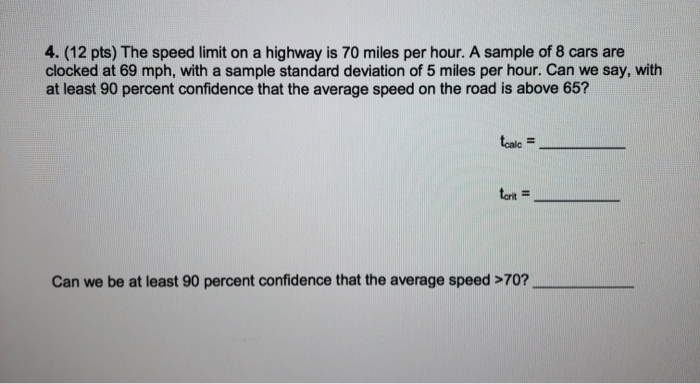4. (12 pts) The speed limit on a highway is 70 miles per hour. A sample of 8 cars are clocked at 69 mph, with a sample standard deviation of 5 miles per hour. Can we say, with at least 90 percent confidence that the average speed on the road is above 65? tcale = tarit Can we be at least 90 percent confidence that the average speed >70?

• ### Statistics, 9e STATISTICS (MAT 127 tice Assignment Gradebook ORION Downloadable eTextbook ment BACK Chapter 06, Section...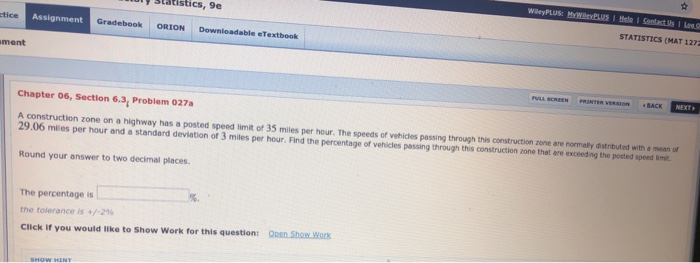Statistics, 9e STATISTICS (MAT 127 tice Assignment Gradebook ORION Downloadable eTextbook ment BACK Chapter 06, Section 6.3, Problem 027a A construction zone on a highway has a posted speed limit of 35 miles per hour. The speeds of vehicles passing through this construction zone are normally distnbuted with a mean of 29.06 mles per hour and a standard devilation of 3 miles per hour. Find the percentage of vehicles passing through this construction zone that are exceeding the posted speed...

• ### The speeds of vehicles on a highway with speed limit 90 km/h are normally distributed with...

The speeds of vehicles on a highway with speed limit 90 km/h are normally distributed with mean 104 km/h and standard deviation 6 km/h. (Round your answers to two decimal places.) (a) What is the probability that a randomly chosen vehicle is traveling at a legal speed? _______ % (b) If police are instructed to ticket motorists driving 110 km/h or more, what percentage of motorist are targeted? _______  %

• ### The speeds of vehicles on a highway with speed limit 110 km/h are normally distributed with...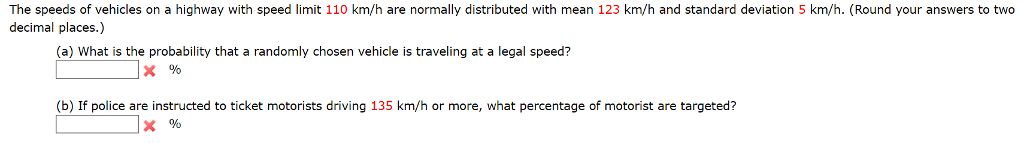The speeds of vehicles on a highway with speed limit 110 km/h are normally distributed with mean 123 km/h and standard deviation 5 km/h. (Round your answers to two decimal places.) (a) What is the probability that a randomly chosen vehicle is traveling at a legal speed? (b) If police are instructed to ticket motorists driving 135 km/h or more, what percentage of motorist are targeted?

• ### The posted speed limit on many US highways is 65 miles per hour

1. The posted speed limit on many US highways is 65 miles per hour. What is this speed in feet per second? 2. 3(3/4) yd is equal to how many inches?

• ### 1. Consider the following sample spot speed data. The speeds are in miles per hour Speed...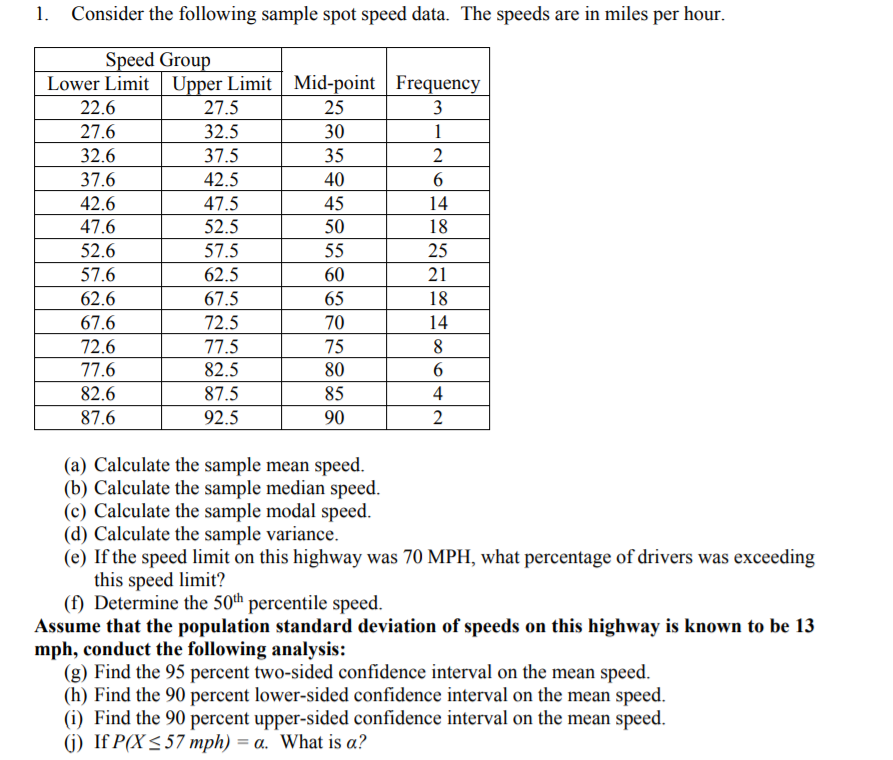1. Consider the following sample spot speed data. The speeds are in miles per hour Speed Grou Lower Limit Upper Limit Mid-point Frequenc 27.5 32.5 37.5 42.5 47.5 52.5 57.5 62.5 67.5 72.5 77.5 82.5 87.5 92.5 25 30 35 40 45 50 22.6 27.6 32.6 37.6 42.6 47.6 52.6 57.6 62.6 67.6 72.6 77.6 82.6 87.6 2 14 25 60 65 70 75 80 85 90 4 (a) Calculate the sample mean speed (b) Calculate the sample median speed...

• ### Use the Empirical Rule. The mean speed of a sample of vehicles along a stretch of...

Use the Empirical Rule. The mean speed of a sample of vehicles along a stretch of highway is 7171 miles per​ hour, with a standard deviation of 33 miles per hour. Estimate the percent of vehicles whose speeds are between 6868 miles per hour and 7474 miles per hour.​ (Assume the data set has a​ bell-shaped distribution.)

• ### Use the Empirical Rule. The mean speed of a sample of vehicles along a stretch of...

Use the Empirical Rule. The mean speed of a sample of vehicles along a stretch of highway is 65miles per​ hour, with a standard deviation of 3 miles per hour. Estimate the percent of vehicles whose speeds are between 56 miles per hour and 74 miles per hour. (Assume the data set has a​ bell-shaped distribution.)